﻿ D2D网络中基于组合拍卖的无线资源分配机制文章快速检索 高级检索
 重庆邮电大学学报(自然科学版)2018, Vol. 30Issue (6): 731-738  DOI: 10.3979/j.issn.1673-825X.2018.06.0010

### 引用本文LI Fangwei, HUANG Xu, ZHANG Haibo, LIU Kaijian. Radio resource allocation mechanism based on combination auction in D2D networks[J]. Journal of Chongqing University of Posts and Telecommunications (Natural Science Edition), 2018, 30(6): 731-738. DOI: 10.3979/j.issn.1673-825X.2018.06.001.### 文章历史

D2D网络中基于组合拍卖的无线资源分配机制

Radio resource allocation mechanism based on combination auction in D2D networks
Chongqing Key Laboratory of Mobile Communications Technology, Chongqing University of Posts and Telecommunications, Chongqing 400065, P. R. China
Foundation Items: The National Natural Science Foundation of China (61271260, 61301122)
Abstract: Aiming at the interference problem of device-to-device (D2D) users and cellular shared spectrum resources, aiming at optimizing the throughput of D2D links in the system as the optimization goal, a resource allocation mechanism for joint power control and channel allocation is proposed. Firstly, according to the interference threshold of D2D users and the signal to interference plus noise ratio (SINR) of cellular users, a multiplexing criterion based on the distance between users is proposed to determine the set of channel resources that can be reused by D2D users. Secondly, under the premise that a given D2D user multiplexes any set of resources, the D2D user's transmit power is adjusted to measure the throughput of each D2D user on different channel resource sets, but power is not allocated temporarily. Finally, based on the result of power control, the combination is adopted. The auction method allocates channels and corresponding transmit power for the D2D link, thereby implementing joint power control and channel allocation, so the system resource allocation result is more reasonable. The simulation results show that this mechanism can effectively suppress the cross-layer interference and the same-layer interference, improve the throughput of D2D links, and enhance the user's QoS.
Keywords: device-to-device (D2D)    throughput    joint resource allocation    water injection algorithm    auction algorithm
0 引言

1 系统模型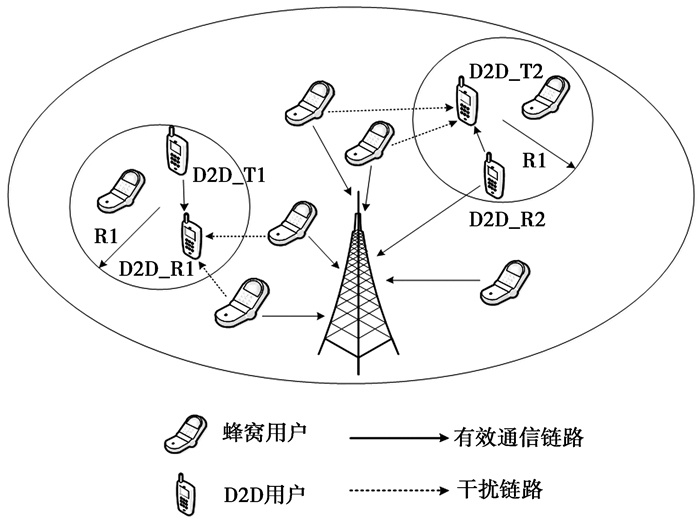图 1 系统模型 Figure 1 System model

 ${h_{{\rm{cd}}}} = k \cdot {\delta _{{\rm{ctd}}}} \cdot {\zeta _{{\rm{ctd}}}} \cdot d_{{\rm{ctd}}}^{ - \alpha }$ (1)

(1) 式中：kα分别表示路径损耗常系数和路径损耗指数；dctd表示蜂窝用户和D2D用户接收端之间的距离；δctdζctd分别表示服从指数分布的快衰落因子和服从对数正态分布的慢衰落因子。

 $\gamma _k^i = \frac{{P_k^i{h_{{\rm{cb}}}}}}{{P_d^i{h_{{\rm{dc}}}} + {N_0}}}$ (2)

D2D用户d的信干噪比为

 $\gamma _d^i = \frac{{P_d^i{h_{{\rm{dd}}}}}}{{P_k^i{h_{{\rm{cd}}}} + {N_0}}}$ (3)

(2)—(3)式中：PkiPdi分别表示蜂窝用户i的发射功率和D2D用户d在蜂窝信道i上发射功率；N0为系统加性高斯白噪声；hcb表示蜂窝用户到基站的信道增益；hdc表示D2D用户发送端到基站的信道增益；hdd表示D2D对之间的信道增益；hcb表示蜂窝用户到D2D用户接收端的信道增益。

C个信道可以组合成N个资源包, 每个资源包中至少含有一条信道资源。当D2D用户d复用第k个资源包Sk，D2D链路的系统容量为

 $u_d^k = \sum\limits_{i \in {S_k}} {{\rm{lb}}\left( {1 + \gamma _d^i} \right)} = \sum\limits_{i \in {S_k}} {{\rm{lb}}\left( {1 + \frac{{P_d^i{h_{{\rm{dd}}}}}}{{P_k^i{h_{{\rm{cd}}}} + {N_0}}}} \right)}$ (4)

 $\max \sum\limits_{k = 1}^{{S_k}} {\sum\limits_{d = 1}^D {{\chi _d}\left( k \right)u_d^k} }$ (5)
 ${\rm{s}}.{\rm{t}}.\;\;r_k^i \ge {R_{c,\min }},k \in \left( {1,2, \cdots ,C} \right)$ (6)
 $r_d^i \ge {R_{d,\min }},d \in \left( {1,2, \cdots ,D} \right)$ (7)
 $\sum\limits_{i \in {S_k}} {P_d^i} \le {P_{c,\max }}$ (8)
 $\sum\limits_{k = 1}^{{S_k}} {{\chi _d}\left( k \right)} \le 1,d = 1,2, \cdots ,D$ (9)
 $\sum\limits_{d = 1}^D {{\chi _d}\left( k \right)} \le 1,k = 1,2, \cdots ,{S_k}$ (10)

(5)—(6)式中：(5)式为最大化系统中D2D链路吞吐量；(6)式和(7)式表示蜂窝用户和D2D用户的最小速率约束；(8)式表示D2D用户的最大发射功率限制；(9)式表示一对D2D用户至多得到一个信道资源集合；(10)式表示一个资源集合至多被分配一次。

2 资源分配

2.1 D2D用可复用信道资源集合

 ${P_{c,\max }}{h_{{\rm{cd}}}} \le {I_{c,d}}$ (11)

(11) 式中：Pc, max表示蜂窝用户的最大发射功率；Ic, d表示D2D用户能接受的最大干扰门限。联立(1)式和(11)式可得

 ${d_{{\rm{ctd}}}} \ge {\left( {\frac{{{P_{c,\max }} \cdot k \cdot {\delta _{{\rm{ctd}}}} \cdot {\zeta _{{\rm{ctd}}}}}}{{{I_{c,d}}}}} \right)^{\frac{1}{\alpha }}} = R$ (12)

2.2 功率控制

 $\max u_d^k = \sum\limits_{i \in {S_k}} {{\rm{lb}}\left( {1 + \frac{{P_d^i{h_{{\rm{dd}}}}}}{{P_k^i{h_{{\rm{cd}}}} + {N_0}}}} \right)}$ (13)
 $0 < P_d^i \le {P_{c,\max }}$ (14)
 $\sum\limits_{i \in {S_k}} {P_d^i \le {P_{c,\max }}}$ (15)

 $\begin{array}{l} L\left( {P_d^i,\alpha ,\beta } \right) = \sum\limits_{i \in {S_k}} {{\rm{lb}}\left( {1 + \frac{{P_d^i{h_{{\rm{dd}}}}}}{{P_k^i{h_{{\rm{cd}}}} + {N_0}}}} \right)} - \\ \;\;\;\;\;\;\;\;\;\;\;\;\;\;\;\;\;\;\;\;\alpha \left( {P_d^i - {P_{c,\max }}} \right) - \beta \left( {\sum\limits_{i \in {S_k}} {P_d^i - {P_{c,\max }}} } \right) \end{array}$ (16)
 $\begin{array}{l} L\left( {P_d^i,\alpha ,\beta } \right) = \sum\limits_{i \in {S_k}} {{\rm{lb}}\left( {1 + \frac{{P_d^i{h_{{\rm{dd}}}}}}{{P_k^i{h_{{\rm{cd}}}} + {N_0}}}} \right)} - \\ \;\;\;\;\;\;\;\;\;\;\;\;\;\;\;\;\;\;\;\;\alpha \left( {P_d^i - {P_{c,\max }}} \right) - \beta \left( {\sum\limits_{i \in {S_k}} {P_d^i - {P_{c,\max }}} } \right) \end{array}$ (17)

(17) 式中，αβ是拉格朗日因子，αβ为常数。计算偏导∂L(Pdi, α, β)/∂Pdi=0得

 $P_d^i = {\left[ {\delta - \frac{1}{H}} \right]^ + }$ (18)

(18) 式中：[φ]+=max{0, φ}；H= $\frac{{{h}_{\text{dd}}}}{P_{k}^{i}{{h}_{\text{cd}}}+{{N}_{0}}}$。很显然，D2D用户的功率分配是多级注水功率分配，δ=1/(α+β)ln2为注水线。因此，可求得任意D2D用户复用任意资源集合Sk时最大的吞吐量及其对应的发射功率Sd, k(udk, Pdi)。

2.3 信道分配

 $V\left( {i,k} \right) = u_d^k$ (19)

 ${P_d}\left( k \right) = \vartheta \sum\limits_{i \in {S_k}} {p_d^i}$ (20)

(20) 式中：ϑ表示单位功率的价格；pdi表示D2D用户d在复用信道资源i时的最优功率分配；Pd(k)表示D2D用户d使用资源集合k时的成本。因此，竞拍者的收益，即它对信道的满意程度可以表示为

 ${U_d}\left( k \right) = V\left( {i,k} \right) - {P_d}\left( k \right)$ (21)

 $\max \sum\limits_{k = 1}^{{S_k}} {\sum\limits_{k = 1}^D {{\chi _d}\left( k \right){U_d}\left( k \right)} }$ (22)

(22) 式中：

 $\sum\limits_{d = 1}^D {{\chi _d}\left( k \right)} \le 1,k = 1,2, \cdots ,{S_k}$ (23)
 $\sum\limits_{k = 1}^{{S_k}} {{\chi _d}\left( k \right)} \le 1,d = 1,2, \cdots ,D$ (24)

3 算法分析 3.1 激励相容性分析

3.2 算法收敛性分析

3.3 算法复杂度分析

4 仿真分析表 1 仿真参数 Table 1 Simulation parameters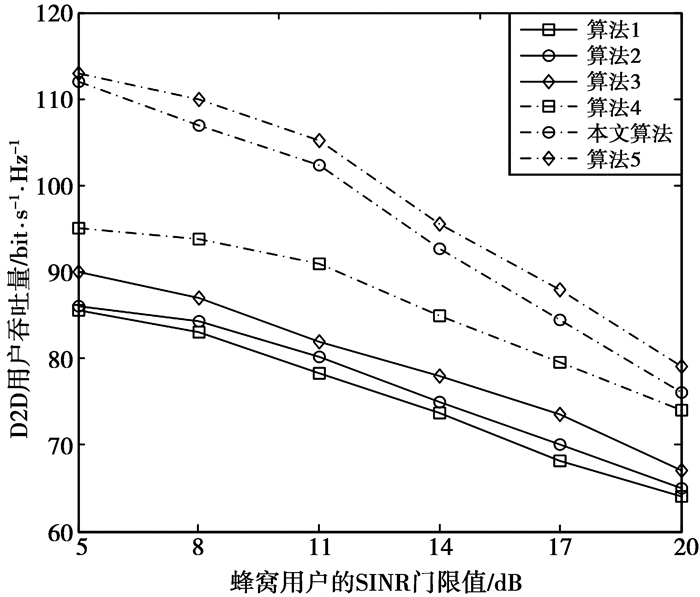图 2 不同SINR门限下D2D用户吞吐量 Figure 2 Throughput of D2D users with different SINR thresholds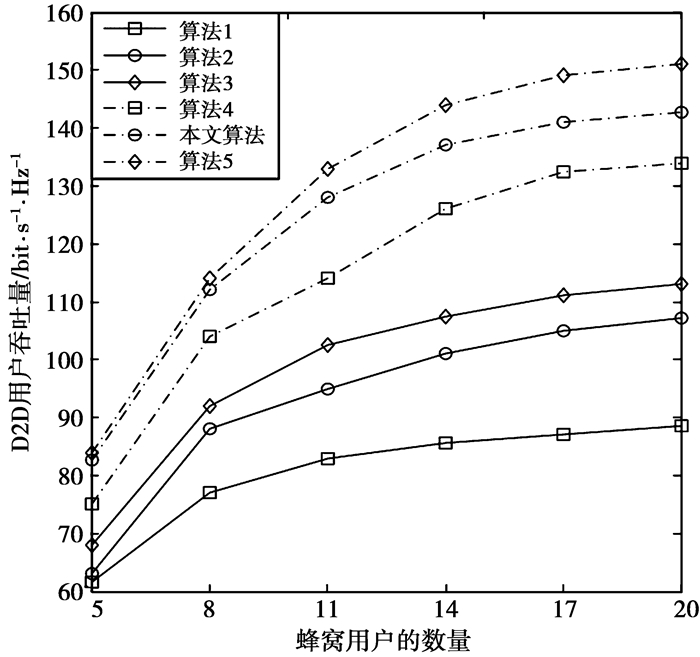图 3 不同蜂窝用户数量下D2D用户吞吐量 Figure 3 Throughput of D2D users with different cellular users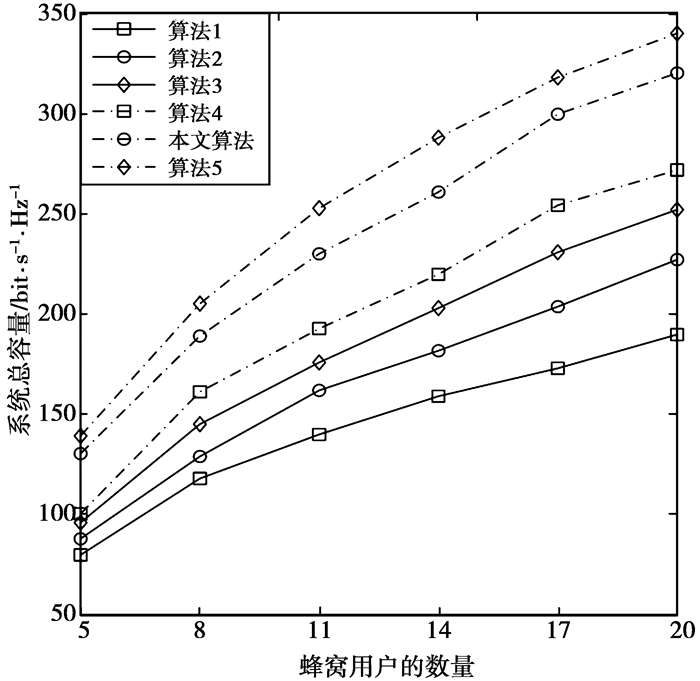图 4 不同蜂窝用户数量下系统总容量 Figure 4 Total system capacity under different number of cellular users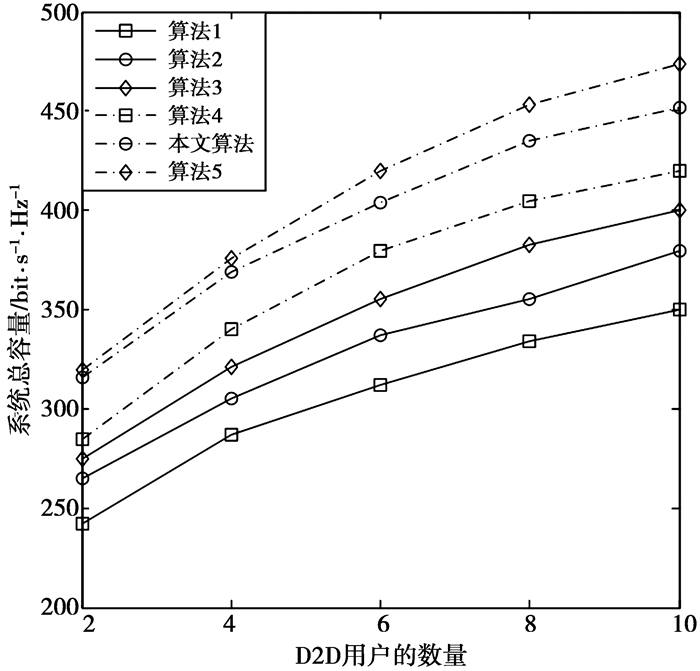图 5 不同D2D用户数量下系统总容量 Figure 5 Total system capacity with different number of D2D users
5 结束语

  MARSCH P, SILVA I D, BULAKCI O, et al. 5G Radio Access Network Architecture: Design Guidelines and Key Considerations[J]. IEEE Communications Magazine, 2016, 54(11): 24-32. DOI:10.1109/MCOM.2016.1600147CM  HUANG Hongcheng, ZHANG Jie, ZHANG Zufan, et al. Interference-Limited device-to-device multi-user cooperation Scheme for optimization of edge networking[J]. Journal of Computer Science and Technology, 2014, 31(6): 1096-1109.  TEHANI M N, UYSAL M, YANIKOMEROGLU H. Device-to-device communication in 5G cellular networks: challenges, solutions, and future directions[J]. IEEE Communications Magazine, 2014, 52(5): 86-92. DOI:10.1109/MCOM.2014.6815897  ZHANG Hongliang, LIAO Yun, SONG Lingyang. D2D-U:Device-to-Device Communications in Unlicensed Bands for 5G System[J]. IEEE Transactions on Wireless Communications, 2015, 14(6): 3505-3519.  CAO Bin, LI Yun, WANG Chonggang. Resource Allocation in Software Defined Wireless Networks[J]. IEEE Networks, 2017, 31(1): 44-51. DOI:10.1109/MNET.2016.1500273NM  MA R, CHANG Y J, CHEN H H, et al.On Relay Selection Schemes for Relay-Assisted D2D Communications in LTE-A Systems[C]//IEEE Transactions on Vehicular Technology. New York: IEEE Press, 2017: 8303-8314. http://ieeexplore.ieee.org/document/7878672/  SWETHA G D, MURTHY G R. Fair resource allocation for D2D communication in mmwave 5G networks[C]//2017 16th Annual Mediterranean Ad Hoc Networking Workshop (Med-Hoc-Net).New York: IEEE Press, 2017: 1-6. https://ieeexplore.ieee.org/document/8001654/  AFZAL A, FEKI A, DEBBAH M, et al. Leveraging D2D Communication to Maximize the Spectral Efficiency of Massive MIMO Systems[C]//2017 15th International Symposium on Modeling and Optimization in Mobile, Ad Hoc, and Wireless Networks (WiOpt). New York: IEEE Press, 2017: 1-6.  MA Ruofei, XIA Nian, CHEN Hsiao Hwa, et al. Mode Selection, Radio Resource Allocation, and Power Coordination in D2D Communications[J]. IEEE Wireless Communications, 2017, 3(24): 112-121.  LI Yun, ZHANG Le, TAN Xin, CAO Bin. An Advanced Spectrum Allocation Algorithm for The Across-cell D2D Communication in LTE Network with Higher Throughput[J]. China Communications, 2016, 13(4): 30-37. DOI:10.1109/CC.2016.7464120  YE Q, AL-SHALASH M, CARAMANIS C, et al. Distributed Resource Allocation in Device-to-Device Enhanced Cellular Networks[J]. Communications IEEE Transactions on, 2014, 63(2): 441-454.  ZHANG Rongqing, SONG Lingyang, HAN Zhu, et al. Distributed resource allocation for device-to-device communications underlaying cellular networks[C]//2013 IEEE International Conference on Communications (ICC). New York: IEEE Press, 2013: 889-1893. http://ieeexplore.ieee.org/xpls/icp.jsp?arnumber=6654797  WEI Lili, HU R.Q, HE Tao, et al. Device-to-device(d2d) communications underlaying MU-MIMO cellular networks[C]//2013 IEEE Global Communications Conference (GLOBECOM). New York: IEEE Press, 2014: 4902-4907. http://ieeexplore.ieee.org/document/6855727/  FODOR G, REIDER N. A Distributed Power Control Scheme for Cellular Network Assisted D2D Communications[C]//2011 IEEE Global Telecommunications Conference(GLOBECOM).New York: IEEE Press, 2012: 1-6. http://ieeexplore.ieee.org/xpls/abs_all.jsp?arnumber=6133537  YU C H, TIRKKONEN O, Doppler K, et al. Power Optimization of Device-to-Device Communication Underlaying Cellular Communication[C]//2009 IEEE International Conference on Communications. New York: IEEE Press, 2009: 1-5. http://ieeexplore.ieee.org/xpls/icp.jsp?arnumber=5199353  ZHONG Wei, FANG Yixin, JIN Shi, et al. Joint Resource Allocation for Device-to-Device Communications Underlaying Uplink MIMO Cellular Networks[J]. IEEE Journal on Selected Areas in Communications, 2015, 33(1): 41-54. DOI:10.1109/JSAC.2014.2369615  ZHU Daohua, WANG Jiaheng, SWINDLEHURST A L, et al. Downlink Resource Reuse for Device-to-Device Communications Underlaying Cellular Networks[J]. IEEE Signal Processing Letters, 2014, 21(5): 531-534. DOI:10.1109/LSP.2014.2309143  FENG Daquan, LU Lu, YI Yuanwu, et al. Device-to-Device Communications Underlaying Cellular Networks[J]. IEEE Transactions on Communications, 2013, 61(8): 3541-3551. DOI:10.1109/TCOMM.2013.071013.120787